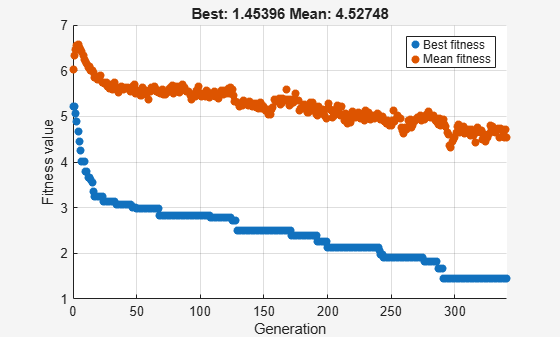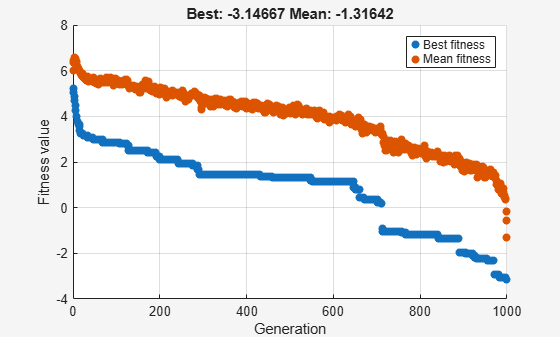# Set Maximum Number of Generations and Stall Generations

The `MaxGenerations` option determines the maximum number of generations the genetic algorithm takes; see Stopping Conditions for the Algorithm. Increasing `MaxGenerations` can improve the final result. The related `MaxStallGenerations` option controls the number of steps `ga` looks over to see whether it is making progress. Increasing `MaxStallGenerations` can enable `ga` to continue when the algorithm needs more function evaluations to find a better solution.

For example, optimize `rastriginsfcn` using 10 variables with default parameters. To observe the solver's progress as it approaches the minimum value of 0, optimize the logarithm of the function.

```rng default % For reproducibility fun = @(x)log(rastriginsfcn(x)); nvar = 10; options = optimoptions('ga','PlotFcn',"gaplotbestf"); [x,fval] = ga(fun,nvar,[],[],[],[],[],[],[],options)```
```Optimization terminated: average change in the fitness value less than options.FunctionTolerance. ``````x = 1×10 -0.0495 -0.0670 -0.0485 0.0174 -0.0087 0.0275 -0.0383 0.0620 -1.0047 -0.0298 ```
```fval = 1.4540 ```

As `ga` approaches the optimal point at the origin, it stalls. To obtain a better solution, set the stall generation limit to 500 and the generation limit to 1000.

```options = optimoptions(options,'MaxStallGenerations',500,'MaxGenerations',1000); rng default % For reproducibility [x,fval] = ga(fun,nvar,[],[],[],[],[],[],[],options)```
```Optimization terminated: maximum number of generations exceeded. ``````x = 1×10 0.0025 -0.0039 0.0021 -0.0030 -0.0053 0.0033 0.0080 0.0012 0.0006 0.0088 ```
```fval = -3.1467 ```

This time the solver approaches the true minimum much more closely.

Watch now